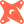Introducing PopSQL + dbt. The first SQL editor with built-in dbt support.Read more →

# How to Get the First Row per Groupin Redshift

Let's say we want to see the first order for every customer for a certain time period. This means we need to order the orders for every customer first. You can use `row_number()` for this:

``````SELECT
*,
row_number() OVER (PARTITION BY customer_id ORDER BY orderdate ASC) AS row_number
FROM lineorder
WHERE orderdate BETWEEN '2018-11-01' AND '2018-11-30';``````

This gives the orders for the time period, plus the `row_number` that resets for each customer. Since we only want to see the first order for each customer, we only want rows that have `row_number = 1`. So we can include this in either a subquery, or better yet a common table expression.:

``````WITH orders AS (
SELECT
*,
row_number() OVER (PARTITION BY customer_id ORDER BY orderdate ASC) AS row_number
FROM lineorder
WHERE orderdate BETWEEN '2018-11-01' AND '2018-11-30'
)
SELECT * FROM orders WHERE row_number = 1;``````

# Ready for a modern SQL editor?